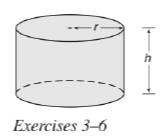Chapter 9.3, Problem 4E### Elementary Geometry for College St...

6th Edition
Daniel C. Alexander + 1 other
ISBN: 9781285195698

#### Solutions

Chapter
Section### Elementary Geometry for College St...

6th Edition
Daniel C. Alexander + 1 other
ISBN: 9781285195698
Textbook Problem
1 views

# For the right circular cylinder shown on page 417, r = 1.75 in. and h = 4.23 in. Find the approximate volume of the cylinder.To determine

To calculate:

The approximate volume of the cylinder.

Explanation

Given:

For a right circular cylinder r=1.75 in and h=4.23 in.

Formula used:

The volume of the right circular cylinder is V=πr2h.

Calculation:

Substitute the known values in the formula,

V=πr2h=π(1

### Still sussing out bartleby?

Check out a sample textbook solution.

See a sample solution

#### The Solution to Your Study Problems

Bartleby provides explanations to thousands of textbook problems written by our experts, many with advanced degrees!

Get Started

#### Give another name for ABC not shown.

Elementary Geometry For College Students, 7e

#### Subtract: (10)(6)

Elementary Technical Mathematics

#### True or False: is a convergent series.

Study Guide for Stewart's Multivariable Calculus, 8th